# Imaginary numbers

Find two imaginary numbers whose sum is a real number. How are the two imaginary numbers related?
What is its sum?

Sum =  0

### Step-by-step explanation:

Answer is a conjugate pair of imaginary numbers (its real parts is zero).
The two imaginary numbers that add up to a real number would be ni and -ni, because ni + (-ni) = ni - ni = 0; where 'n' is any real number, no zero. Thus, the sum of this two imaginary numbers become a real number 0.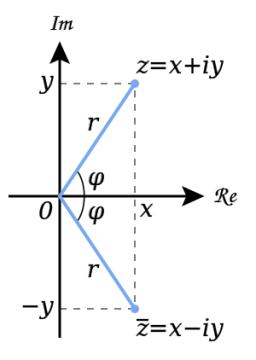Did you find an error or inaccuracy? Feel free to write us. Thank you!

Showing 1 comment:Dr Math
add up a number and its conjugateTips to related online calculators

## Related math problems and questions:

• Distance two imaginary numbs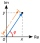Find the distance between two complex number: z1=(-8+i) and z2=(-1+i).
• Conjugate equation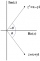Find the numbers of a and b, if (a - bi) (3 + 5i) is the Conjugate of (-6 - 24i)
• Linear imaginary equationGiven that 2(z+i)=i(z+i) "this is z star" Find the value of the complex number z.
• Evaluate 18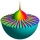Evaluate the expression (-4-7i)-(-6-9i) and write the result in the form a+bi (Real + i* Imaginary).
• De Moivre's formula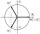There are two distinct complex numbers z, such that z3 is equal to 1 and z is not equal to 1. Calculate the sum of these two numbers.
• An Argand diagram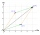Find the sum z1+z2 and difference z1-z2 and z2-z1 of z1=5+2i and z2=2+3i by an Argand diagram.
• The modulus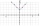Find the modulus of the complex number 2 + 5i
• The sum 10The sum of three decimals are 938.629. Two of them are 456.54 and 392.69. Find the third one.
• Is complexAre these numbers 2i, 4i, 2i + 1, 8i, 2i + 3, 4 + 7i, 8i, 8i + 4, 5i, 6i, 3i complex?
• Three numbersFind three numbers so that the second number is 4 times greater than the first and the third is lower by 5 than the second number. Their sum is 67.
• Find the sumFind the sum of all natural numbers from 1 and 100, which are divisible by 2 or 5To three-eighths of one third, we add five quarters of one half and multiply the sum by four. How much will we get?Sum of the two numbers is 15 1/6. If one of the numbers is 2 2/3, find the other number.If z1=5+3i and z2=4-2i, write the following in the form a+bi a) 4z1+6z2 b) z1*z2We have three different non-zero digits. We will create all 3 digits numbers from them to use all 3 figures in each number. We add all the created numbers, and we get the sum of 1554. What were the numbers?Find the sum of all odd integers from 13 to 781.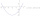The sum of of three numbers is 106. the first number is 10 more then the third. The second number is 2x the third. What are the numbers?